Next: Rational numbers Up: Signature of unknowns Previous: Signature of unknowns

### Finding simple relations among consecutive polynomial values

The above relationship,

p(x+1)+p(x+2)+p(x+6) = p(x)+p(x+4)+p(x+5)

is a relation that will hold for any polynomial of degree 2. It is interesting as a side topic to be able to generate this kind of simple relationships, not just for coefficients 1 and -1, but for small coefficients. This can be resolved with signatures of expressions and the LLL lattice reduction algorithm, and it is a very good example of both. The problem can be formalized as follows: we are looking for a polynomial p(x) = adxd+...a1x+a0such that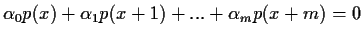for any coefficients in p(x) and for any value of x. Furthermore, we are interested in the case where the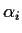coefficients are small.

For this we will construct thematrix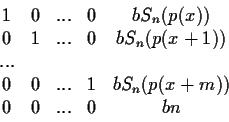where b is an integer blow up factor, used to make sure that for the shortest vectors, a 0 will be in that column, and hence the corresponding relations with the polynomials are exact. The last column contains all the signatures of the unknown polynomial, which are computed (mod n). The last row serves the purpose of computing the linear combinations of the signatures (mod n).

For example, if we let d=2, n=100000007, m=6 and b=10 and the random variables assigned to the unknowns:and x=56110369 then the matrix is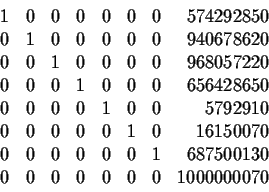Applying the LLL algorithm to the above produces the reduced matrix: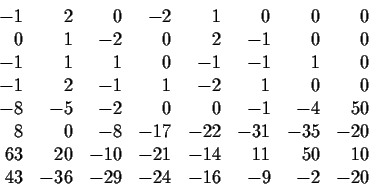From these short vectors we can conclude the previous relation from row 3 and the relation

2p(x+1)+p(x+4) = p(x)+2p(x+3)

from row 1. These relations can be verified with a computer algebra system if necessary.Next: Rational numbers Up: Signature of unknowns Previous: Signature of unknowns
Gaston Gonnet
1999-07-04